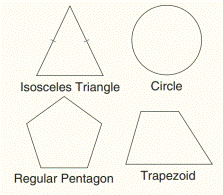Chapter 2.CR, Problem 44CR### Elementary Geometry for College St...

6th Edition
Daniel C. Alexander + 1 other
ISBN: 9781285195698

#### Solutions

Chapter
Section### Elementary Geometry for College St...

6th Edition
Daniel C. Alexander + 1 other
ISBN: 9781285195698
Textbook Problem
5 views

# Which figures have a ) line symmetry (at least one axis)? b ) point symmetry?To determine

a)

To find:

The line of symmetry.

Explanation

Given:

The given figures are,

Figure (1)

Definition:

A figure has symmetry with respect to a point P if for every point M on the figure, there is a second point N on the figure for which point P is the midpoint of MN¯.

A figure has symmetry with respect to a line 𝓁 if for every point X on the figure, there is a second point Y on the figure for which 𝓁 is the perpendicular bisector of XY¯.

Approach:

The line of symmetry for isosceles triangle is shown in the following figure (2)

To determine

(b)

To find:

The point of symmetry

### Still sussing out bartleby?

Check out a sample textbook solution.

See a sample solution

#### The Solution to Your Study Problems

Bartleby provides explanations to thousands of textbook problems written by our experts, many with advanced degrees!

Get Started